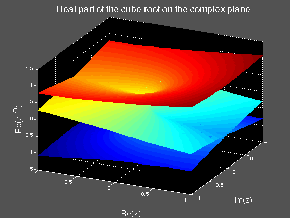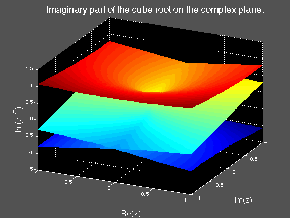18.04 | Fall 1999 | Undergraduate

# Complex Variables with Applications

Study Materials

## Multiple Valued Functions

(All images created with MATLAB® software.)

Click on pictures for more information and an image of higher resolution.

Multiple values are impossible to avoid in the context of complex valued functions. It is a feature present even in some of the most elementary functions (such as the logarithm and the square root). Actually, multiple valued functions in the complex plane give rise to many interesting and intriguing phenomena, so perhaps the fact that they cannot be avoided is a blessing in disguise. Multiple values, of course, also arise for real valued functions. But there, quite often, the various values can be cleanly separated into different functions. Thus, one talks of the positive (or the negative) square root and the idea of the logarithm as multiple valued does not even arise!

By contrast, for functions of a complex variable, the various values are usually smoothly “connected”. By moving around in the complex plane and tracking the values of the function, it is possible to switch values after going around a closed path. Thus, it is not possible to split (in some natural/obvious way) the multiple values into different functions. The points around which these connections occur (i.e. where the value of the function switches) are called branch points.Figure 1: Real part of the cube root on the complex plane.

We illustrate this behavior here with the example of the cubic root function on the complex plane. Figure 1 shows the real part of the cubic root and Figure 2 shows the imaginary part. At each point in the complex plane (except for the origin) there are three possible cubic roots and this gives rise to the “three level surfaces” that show in each of the pictures. But these three surfaces are, in fact, not disjoint. Notice how they wrap around the origin (a branch point) so that it is possible to go from one sheet to another simply by moving around the origin.

In fact, the three surfaces are just one nice smooth surface (except at the origin), but to see this we would have to be able to make four dimensional graphs! The crossings that occur in the pictures are similar to the crossings one would see when projecting a perfectly fine single valued curve in three dimensions with (say) a knot, onto the plane.

All of this becomes a bit clearer using the notion of the Riemann Surface associated with a multiple valued function on the complex plane. Below we will give several examples of this idea.Figure 2: Imaginary part of the cube root on the complex plane.